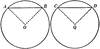# Equal Chords in Equal Circles TheoremIllustration used to show that “In equal circles, or in the same circle, if two chords are equal, they subtend equal arcs; conversely, if two arcs are equal, the chords that subtend them are equal.”

### Keywords

geometry, circle, arc, proof, chord, chords, theorem, equal arcs, prove, theorems, proofs

### Galleries

Geometric Theorems and Proofs

### Source

C.A. Hart, Daniel D. Feldman Plane and Solid Geometry (New York, NY: American Book Company, 1911)

TIFF (full resolution)

2400×1188, 141.4 KiB

Large GIF

1024×506, 22.4 KiB

Medium GIF

640×316, 13.4 KiB

Small GIF

320×158, 6.0 KiB